# Select the best feature using anova for machine learningPosted by

### OUTLINE:

• Introduction
• An important point in ANOVA
• Types of ANOVA
• Calculation of on way ANOVA
• Assumption in ANOVA
• Python example
• Conclusion

## Introduction of ANalysis Of VAriance (ANOVA):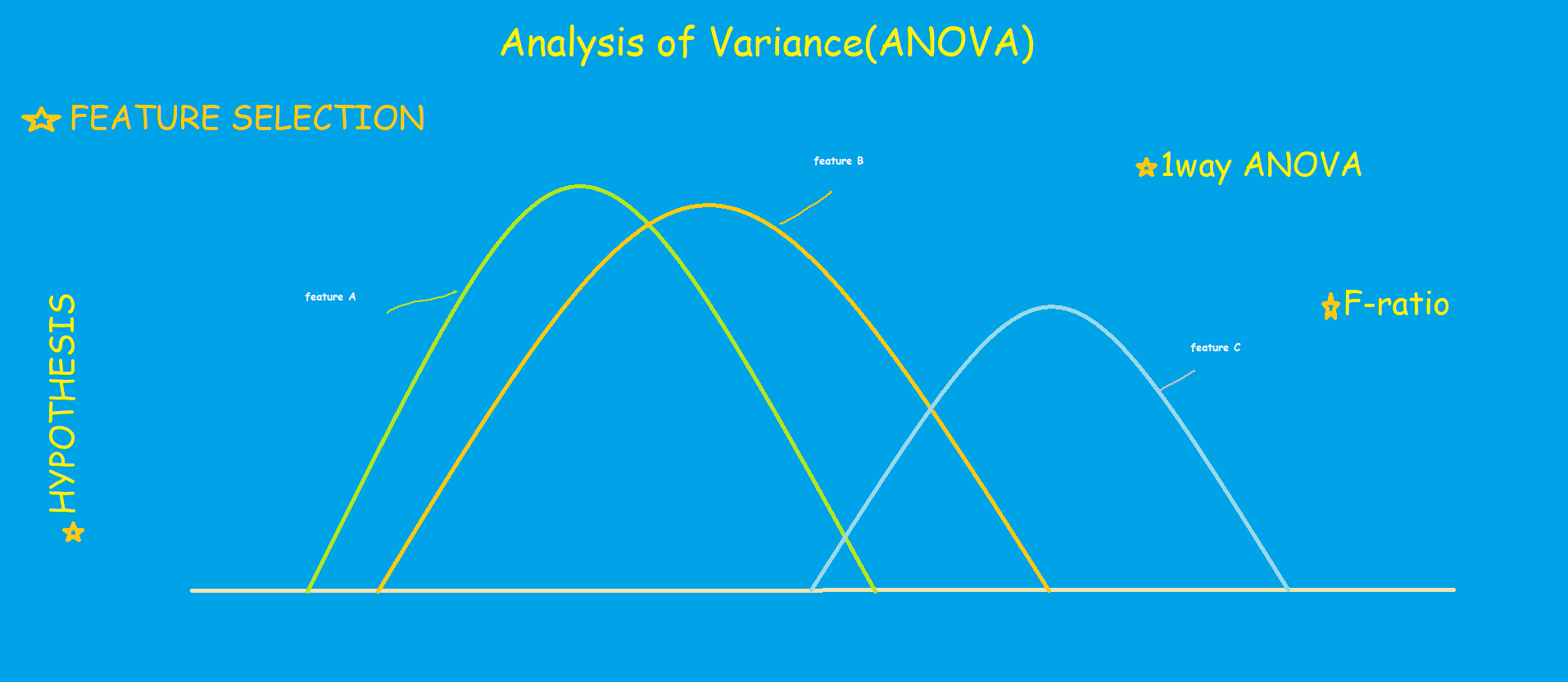Feature selection is one of the most critical tasks in machine learning that greatly impacts the performance of the model. The selection of irrelevant features not only reduces the accuracy of the model but also consumes more resources.

Let’s say we have a high dimensional dateset. The very next challenge will be selecting the best features for the model.

We have to select only those features that are non-correlated among themselves at the same time these features should be highly dependent on the target variable. In other words, we must keep only highly significant features.

In this blog, we shall discuss ANOVA, which reveals the dependency between the  features and the target variable. Therefore, we shall select only those features which are highly dependent on the target variable.## Important points  in ANOVA:

• The response variable, dependent variable, outcome variable, target variable are all same. It is represented as Y in the dataset.
• predictor variable and the independent variable are the same. It is represented as X.
• The Variance is the measurement of the spread between numbers in a variable. In other words, it measures how far a number is from the mean and every number in a variable.
• Null Hypothesis: means of all groups are equal.
• Alternate Hypothesis: at least mean are not equal.
• Grand Mean: mean of the dataset.
• Individual Mean: mean of each feature.
• F-ratio: used to examine if there are any differences between the group in an experiment.

### Calculation of  ANOVA:

Let’s say we have 3 features with 5 samples each.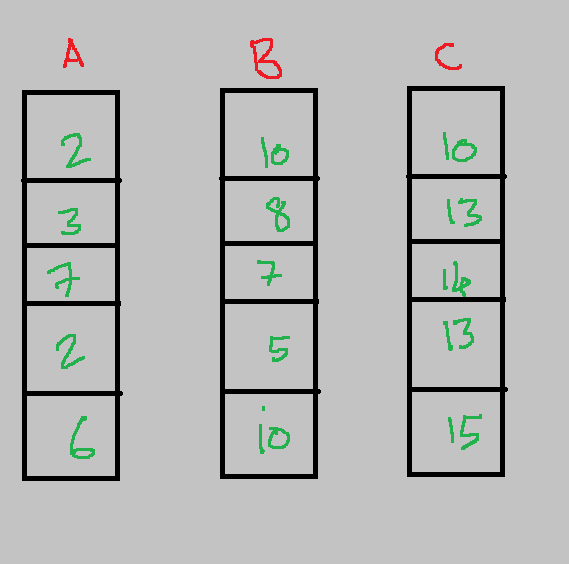Step1: Calculate the mean of the features:Step2: Calculate the sum of squares within the groups. Refer to the below image.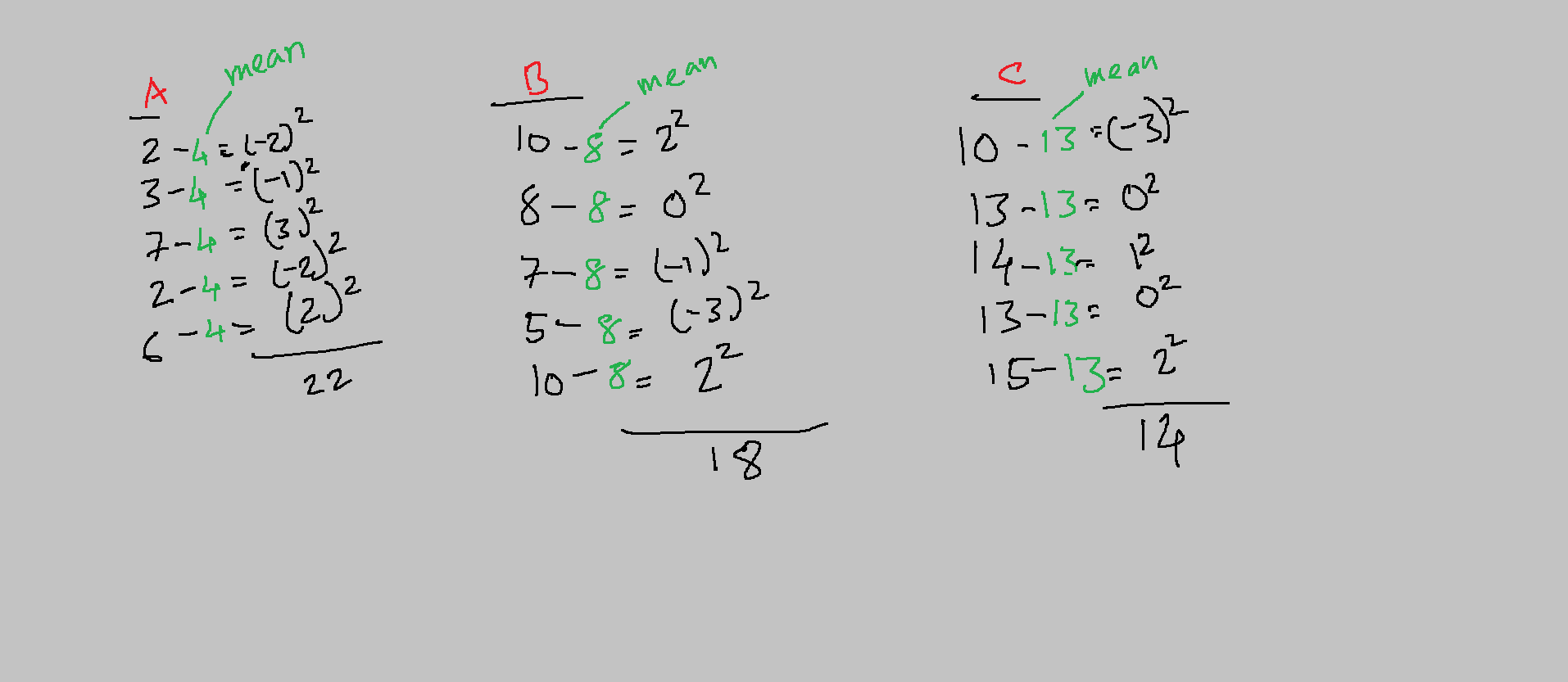Step3: Grand meanStep4: Calculate the total sum of squaresThe total sum of squares, in this case, is 257.3

Step 5: Calculate the sum of the square between the group(features)

Step 6: Getting degrees of freedom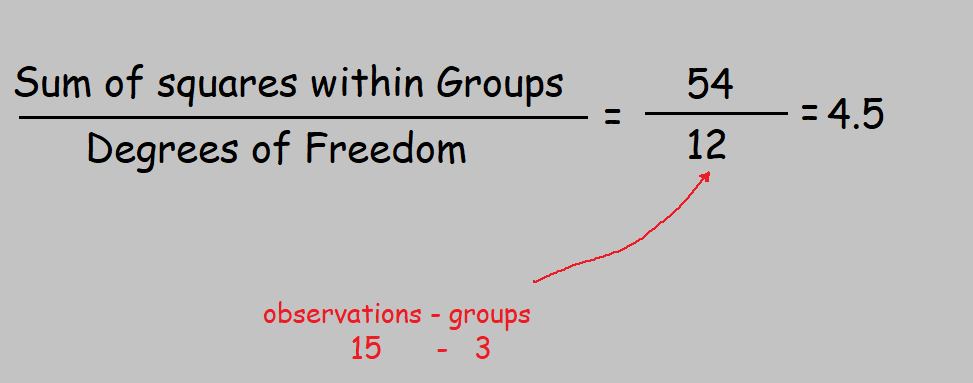The two degrees of freedom i.e 2 and 12 will be used to pick a critical value from the F distribution table.

Step 7: Getting F-ratio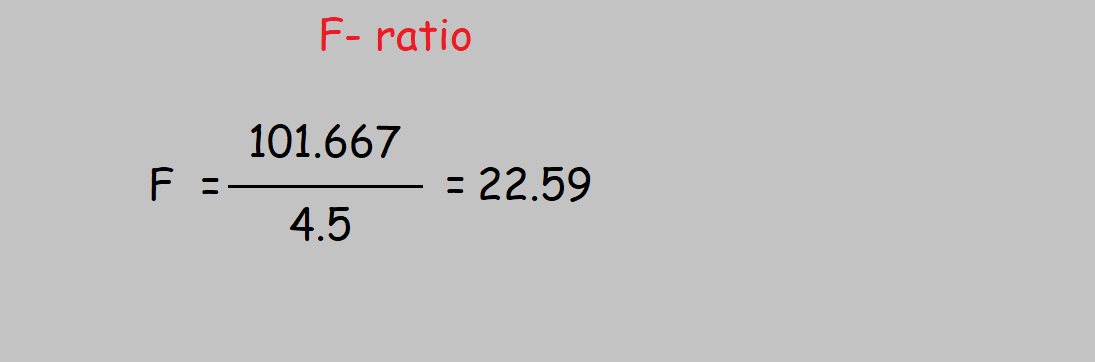Step 8:  When we take 2 and 12 as input, that is F(2,12) with p-value =0.05, we get 3.885, which is also know as critical value.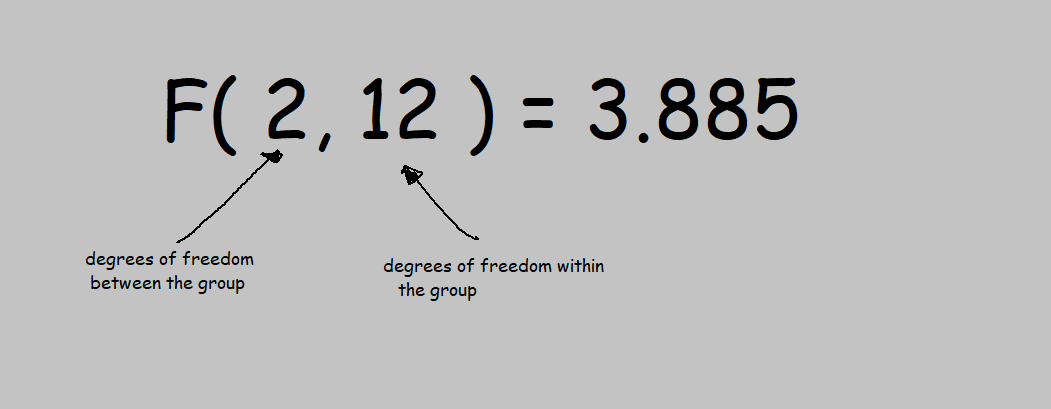As  22.59 falls in the Rejection Region, we reject Null Hypothesis. in other words, we can include this feature in the model training.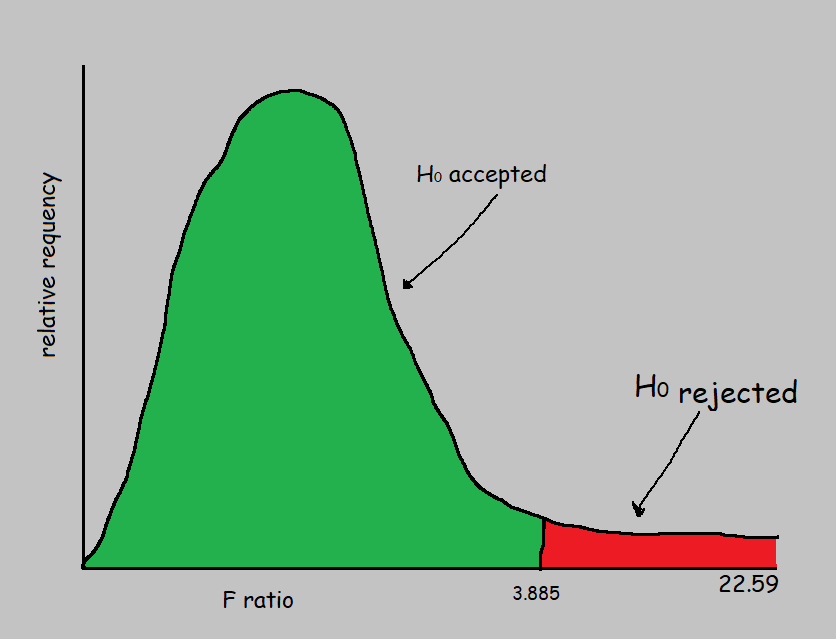• The following python code returns F-ratio(score)
```     import scipy
scipy.stats.f.ppf(q=1-0.05, dfn=2, dfd=12) ```

### Types of ANOVA and facts:

• One-way ANOVA: One independent variable, with three classes and the target variable, is continuous.
• One-way ANOA example 1:  an independent variable that holds “TV brand”(categorical) and it contains three brands. The dependent variable holds the price difference of TV.
• Two-way ANOVA: the primary use of two-way ANOVA is checking whether there is any interaction between two independent variables and the target variable.
• The two independent variables must be categorical and the target variable is continuous.
• Example: let’s say, we want to find out whether there is any interaction between the two independent variable physical activity(low, medium, high), standard of the student(class 8,9,10), and marks obtained by the student which is a continuous variable.

### Few assumptions in ANOVA:

1. The dependent variable should be continuous.
2. The Independent feature should have more than two groups. Example: profession(doctor, software engineer, accountant, businessman, singer).
3. If there are two independent categorical features, groups between them should not be common. Example: “doctor” group should not be present in the second feature.
4. It is highly sensitive towards outliers. The presence of any outliers in the dataset reduces the validity of the result. Therefore, we must remove outliers from the dataset before pushing it to the ANOVA model.
5.  1way ANOVA: the target variable should be normally distributed for the group of the independent variable. 2way ANOVA: The target variable should be normally distributed for each group of the two independent variables.
6. 1way ANOVA: there should be homogeneity of variance between the independent and independent variables. 2way ANOVA: There should be homogeneity of variances for each combination of groups of the two independent features.

Homogeneity of Variances: it is an assumption which says that the distribution between the dependent and independent variable is the same.

### Python example of 2 way ANOVA:

```import numpy as np
import pandas as pd

df = pd.DataFrame({'rainfall': np.repeat(['daily', 'weekly'], 15),'sunlight': np.tile(np.repeat(['low', 'med', 'high'], 5), 2),\
'temperature': [6, 6, 6, 5, 6, 5, 5, 6, 4, 5,6, 6, 7, 8, 7, 3, 4, 4, 4, 5,4, 4, 4, 4, 4, 5, 6, 6, 7, 8]})

import statsmodels.api as sm
from statsmodels.formula.api import ols

model = ols('temperature ~ C(rainfall) + C(sunlight) + C(rainfall):C(sunlight)', data=df).fit()
print(sm.stats.anova_lm(model, typ=2))```

Below is the picture which shows the output of the python code.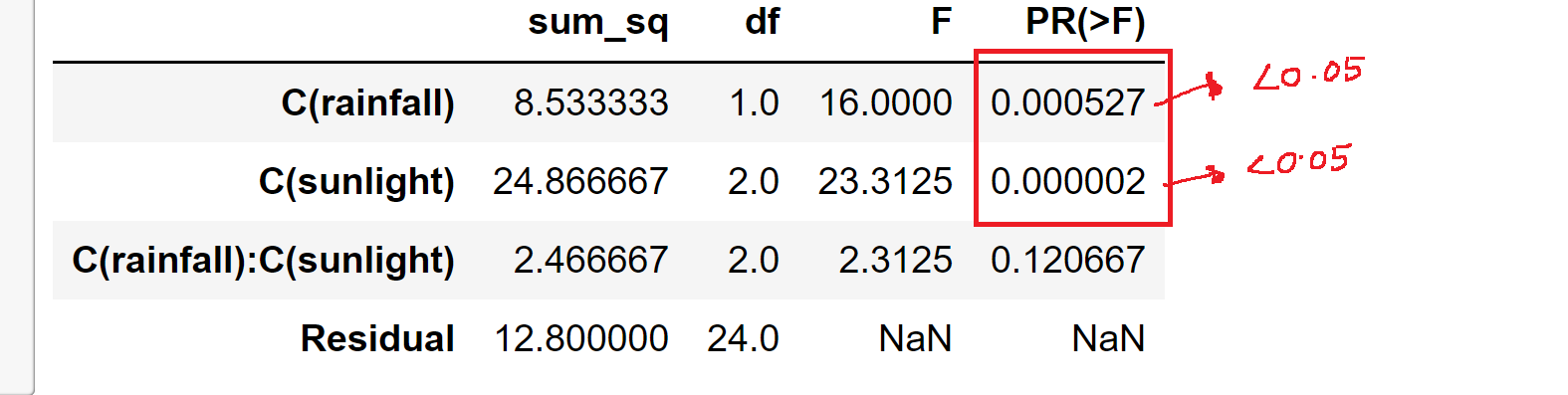Hence, We can observe that the P-value of rainfall and sunlight is less than 0.05, which means that these factors have a high impact on temperature.

### Conclusion:

In this blog, we discussed ANOVA and how it calculates manually. Moreover, We have also covered how it is useful for selecting features.

There are a few assumptions like there should not be outliers in the dataset, independent categorical variables should not have common groups.

We discussed 1-way and 2-way ANOVA. The above python code returns a p-value for each feature which will help us to select only significant features from the dataset.

We hope this article helps you to understand ANOVA at a basic level. If you any questions let us know in the comment section.